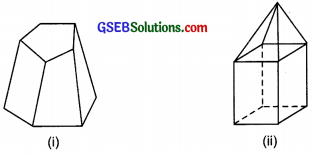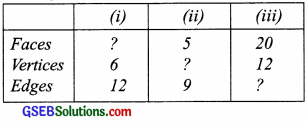# GSEB Solutions Class 8 Maths Chapter 10 Visualizing Solid Shapes Ex 10.3

Gujarat Board GSEB Textbook Solutions Class 8 Maths Chapter 10 Visualizing Solid Shapes Ex 10.3 Textbook Questions and Answers.

## Gujarat Board Textbook Solutions Class 8 Maths Chapter 10 Visualizing Solid Shapes Ex 10.3

Question 1.
Can a polyhedron have four its faces

1. 3 triangles?
2. 4 triangles?
3. a square and four triangles?

Solution:
A polyhedron is bounded by four or more than four polygonal faces.

1. No, it is not possible that a polyhedron has 3 triangles for its faces.
2. Yes, 4 triangles can be the faces of a polyhedron.
3. Yes, a square and 4 triangles can be the faces of a polyhedron.Question 2.
Is it possible to have a polyhedron with any given number of faces?
Hint:
Think of a pyramid.
Solution:
Yes, it can be possible only if the number of faces is four or more than four.

Question 3.
Which are prisms among the following?Solution:
Since, a prism is a polyhedron having two of its faces congruent and parallel, whereas other faces are parallelogram.

1. No, a nail is not a prism.
2. Yes, unsharpened pencil is a prism.
3. No, table weight is not a prism.
4. Yes, box is a prism.Question 4.

1. How are prisms and cylinders alike?
2. How are pyramids and cones alike?

Solution:

1. Both of the prisms and cylinders have their base and top as congruent faces and parallel to each other. Also, a prism becomes a cylinder as the number of sides of its base becomes larger and larger.
2. The pyramid and cones are alike because their lateral faces meet at a vertex. Also a pyramid becomes a cone as the number of sides of its base becomes larger and larger.

Question 5.
Is a square prism same as a cube? Explain?
Solution:
No, not always, because it can be a cuboid also.

Question 6.
Verify Euler’s formula for these solids?Solution:
(i) In figure (i), we have
F = 7, V= 10 and E = 15
∴ F + F = 7 + 10 = 17
F + V – E = 17 – 15 = 2
i.e., F + V – E = 2
Thus, Euler’s formula is verified.

(ii) In figure (ii), we have
F = 9, V = 9 and E = 16
∴ F + V = 9 + 9 = 18 and
F + V – E = 18 – 16 = 2
i ,e., F + V – E = 2
Thus, Euler’s formula is verified.Question 7.
Using Euler’s formula find the unknown?Solution:
1. Here, V = 6 and E = 12
Since F + V – E = 2
∴ F + 6 – 12 = 2 or F – 6 = 2
or F = 2 + 6 = 8

2. Here, F = 5 and E = 9
Since F + V – E = 2 or
∴ 5 + V – 9 = 2
or V – 4 = 2
or V = 2 + 4 = 6

3. Here F = 20 and V = 12
Since, F + V – E = 2
∴ 20 + 12 – E = 2
32 – E = 2
or E = 32 – 2 = 30Question 8.
Can polyhedron have 10 faces, 20 edges and 15 vertices?
Solution:
Here, F = 10, E = 20 and V = 15
We have: F + V – E = 2
∴ 10 + 15 – 20 = 2 or
25 – 20 = 2
or 5 = 2 which is not true
i.e., F + V – E ≠ 2
Thus, such a polyhedron is not possible.